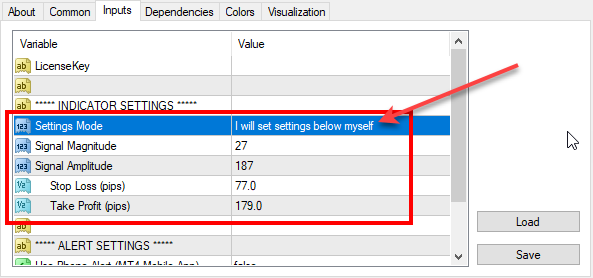# Re-optimized FxMagnetic indicator on GBPJPY (2019-02-09)

We’ve reoptimized FxMagnetic indicator settings on GBPJPY. Here are the latest parameters for all GBPJPY timeframes that you should use for best MT4 indicator performance.To set parameters manually you need to switch the Settings Mode to: I will set settings below myself

Don’t have FxMagnetic indicator yet? You can buy FxMagnetic here.

## 1-minute chart:

Signal Magnitude = 33

Signal Amplitude = 166

Stop Loss (pips) = 28

Take Profit (pips) = 85

## 5-minute chart:

Signal Magnitude = 22

Signal Amplitude = 177

Stop Loss (pips) = 69

Take Profit (pips) = 112

## 15-minute chart:

Signal Magnitude = 30;

Signal Amplitude = 207;

Stop Loss (pips) = 101;

Take Profit (pips) = 203;

## 30-minute chart:

Signal Magnitude = 58;

Signal Amplitude = 203;

Stop Loss (pips) = 74;

Take Profit (pips) = 243;

## 1-hour chart:

Signal Magnitude = 29;

Signal Amplitude = 206;

Stop Loss (pips) = 102;

Take Profit (pips) = 197;

## 4-hour chart:

Signal Magnitude = 14;

Signal Amplitude = 154;

Stop Loss (pips) = 277;

Take Profit (pips) = 464;

## Daily chart:

Signal Magnitude = 40;

Signal Amplitude = 160;

Stop Loss (pips) = 340;

Take Profit (pips) = 540;

## Weekly chart:

Signal Magnitude = 5;

Ind0Param1 = 150;

Stop Loss (pips) = 650;

Take Profit (pips) = 750;

## Monthly chart:

Signal Magnitude = 5;

Signal Amplitude = 140;

Stop Loss (pips) = 700;

Take Profit (pips) = 2800;

## FxMagnetic GBPJPY v1.1 stats on 9th February, 2019

Below are the screenshots of FxMagnetic GBPJPY stats from the previous 100 signals. This was taken on 2019-02-09 on FxOpen Live ECN account. We re-optimized FxMagnetic GBPJPY v1.1 on this broker so you’ll see best signals with them.

## New features in FxMagnetic GBPJPY v1.1 indicator

The new FxMagnetic GBPJPY v1.1 displays the date and time of the oldest signal on the chart. This is very useful because now you will have a clue how much time it took for the FxMagnetic to generate all previous 100 signals on the chart to produce the results.

The new FxMagnetic GBPJPY also displays current signal direction and previous closed signal Profit/Loss figure in the same colors as the arrows on the chart. This helps you spot signal information easier.